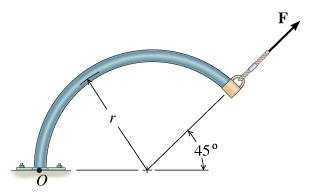# Scalar Moment around a point due to a force

## Homework Statement

In two dimensions, the moment of a force can be calculated using the scalar method, MO=Fd, where F is the magnitude of the force and d is the perpendicular distance from the line of force to the point where the moment is being considered.

Using the scalar method, calculate the moment about O due to F.

## Homework Equations[/B]
r = 2.50 ft and F has magnitude F = 580 lb.

## The Attempt at a Solution

I've calculated the x and y components of the force, each being 410 lb, due to the angle being 45
Also using the angles I calculated the x and y distances being 1.77 and then 1.77 + 2.5?

I do the f*d multiplied and added up for 1.77*410*2 and it's wrong
I then divided that by 2, and it was wrong.
I then did the (2.55+1.77*410)+(1.77*410) and it says that's wrong too.

What am I doing wrong?